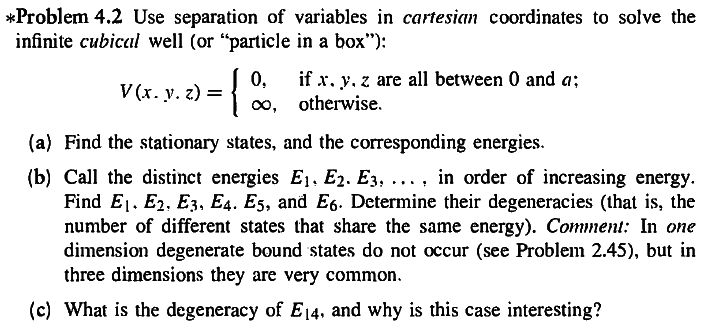# Particle in a box (3D)

Gold Member

## Homework Statement## The Attempt at a Solution

a)
for ##0 \le x,y,z \le a##, the potential is zero. Therefore, the 3d schrödinger equation is

$$- \frac {\hbar^{2}}{2m} \nabla^{2} \psi = E \psi$$
Is it okay to write 3 equations
$$- \frac {\hbar^{2}}{2m} \frac {\partial^{2} \psi}{\partial x^{2}} = E \psi$$
$$- \frac {\hbar^{2}}{2m} \frac {\partial^{2} \psi}{\partial y^{2}} = E \psi$$
$$- \frac {\hbar^{2}}{2m} \frac {\partial^{2} \psi}{\partial z^{2}} = E \psi$$

How do I separate this into 3 second order ODE's?

Last edited:

Orodruin
Staff Emeritus
Homework Helper
Gold Member
How do you usually separate variables?

Orodruin
Staff Emeritus
Homework Helper
Gold Member
How do you usually separate variables?

Gold Member
I don't usually separate variables, that is my problem

Orodruin
Staff Emeritus
Homework Helper
Gold Member
Then I suggest starting with reading up on variable separation. A basic Google search should provide you with reasonable results.

Gold Member
separation of variables to me means something of the following

$$\frac {dy}{dt} = C$$
$$dy = C dt$$
$$y = \int C dt$$

This "separation of variables" seems to be something very different.

Orodruin
Staff Emeritus
Homework Helper
Gold Member
Gold Member
Okay, I know what "separation of variables" is then. However, I didn't know it by that name, or any name for that matter, but at least I now know what you are talking about. So I will say ##\psi(x,y,z) = X(x)Y(y)Z(z)##. Then my schrödinger equation will be

$$- \frac {\hbar^{2}}{2m} \Bigg [ \frac {\partial^{2} (XYZ)}{\partial x^{2}} + \frac {\partial^{2} (XYZ)}{\partial y^{2}} + \frac {\partial^{2} (XYZ)}{\partial z^{2}} \Bigg ] = EXYZ$$
Pull out the constants (i.e. Y and Z are not functions of x, so they can be pulled out of the derivative with respect to x, etc). Divide through by ##XYZ##
$$- \frac {\hbar^{2}}{2m} \Bigg [ \frac {1}{X} \frac {\partial^{2} X}{\partial x^{2}} + \frac {1}{Y} \frac {\partial^{2} Y}{\partial y^{2}} + \frac {1}{Z} \frac {\partial^{2} Z}{\partial z^{2}} \Bigg ] = E$$
Okay, now at this part I have a sum of three second order partial derivatives. How do I proceed here?

jtbell
Mentor
Have you studied how the one-dimensional time-independent Schrödinger equation is derived from the time-dependent SE by using separation of variables (in this case x and t)? That is, starting with $$-\frac{\hbar^2}{2m} \frac{\partial^2}{\partial x^2} \Psi(x,t) + V(x) \Psi(x,t) = i\hbar \frac{\partial}{\partial t} \Psi(x,t)$$ you separate it into two equations, one for x and one for t, namely the time-independent SE $$-\frac{\hbar^2}{2m} \frac {d^2}{dx^2} \psi(x) + V(x) \psi(x) = E \psi(x)$$ and a differential equation for t: $$i\hbar \frac{d}{dt}f(t) = Ef(t)$$ You should review that derivation, or study it if you haven't seen it yet.

jtbell
Mentor
Ah, you wrote your last post while I was writing mine.

OK, the right side of your last equation is a constant, so the left side has to be a constant, the same constant regardless of what values you choose for the coordinates x, y and z, and those coordinates can be chosen completely independently from each other. What does that tell you about the three terms on the left side?

Gold Member
Hi Jtbell,

Yes, I have studied all of the following, however I can't connect the dots with what I am learning now. It is almost like they are completely separate animals...

Gold Member
Anyways, I see that the sum of all these derivatives is equal to a constant. Does that mean each term is a constant?

So maybe for the first derivative, I would set
$$\frac {1}{X} \frac {\partial^{2} X}{\partial x^{2}} = C_{1}$$
And so forth with the rest, then solve each term?

jtbell
Mentor
Anyways, I see that the sum of all these derivatives is equal to a constant. Does that mean each term is a constant?

Yes. A key factor in the reasoning here is that you can choose the three coordinates x, y, z independently. So if you choose x and y, that gives you values for the first two terms (after you've found the functions X, Y, Z of course). These and the value of E tell you what the value of the third term has to be, and it has to have that value regardless of the value of z. You can reason similarly for the other two terms. So each term on the left is a constant, possibly a different constant for each.

So maybe for the first derivative, I would set
$$\frac {1}{X} \frac {\partial^{2} X}{\partial x^{2}} = C_{1}$$
And so forth with the rest, then solve each term?

Right. It might simplify things a bit if you include the ##-\hbar^2/2m## factor in each term. That is, distribute it over the square brackets. The equation you get for each term should then look familiar, especially if you give the constants names like Ex, Ey and Ez instead of C1, C2 and C3.